# Weight in 45 tons Weight out 23 tons

• Slides: 52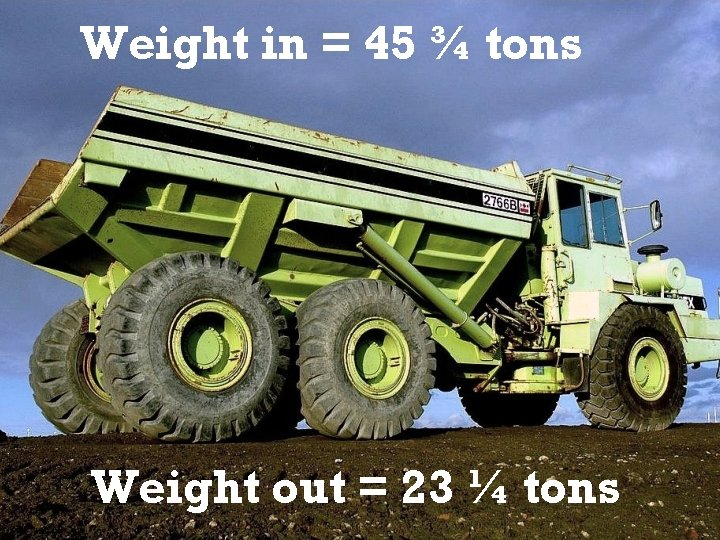Weight in = 45 ¾ tons Weight out = 23 ¼ tonsAt the end of this module, you should be able to subtract fractions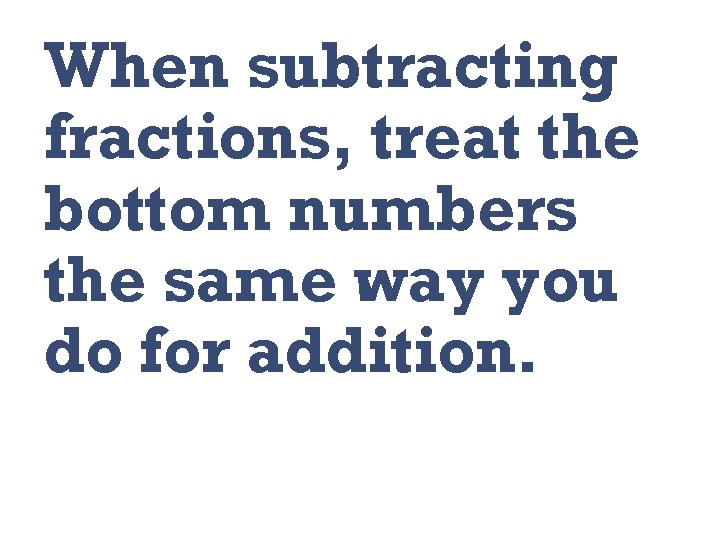When subtracting fractions, treat the bottom numbers the same way you do for addition.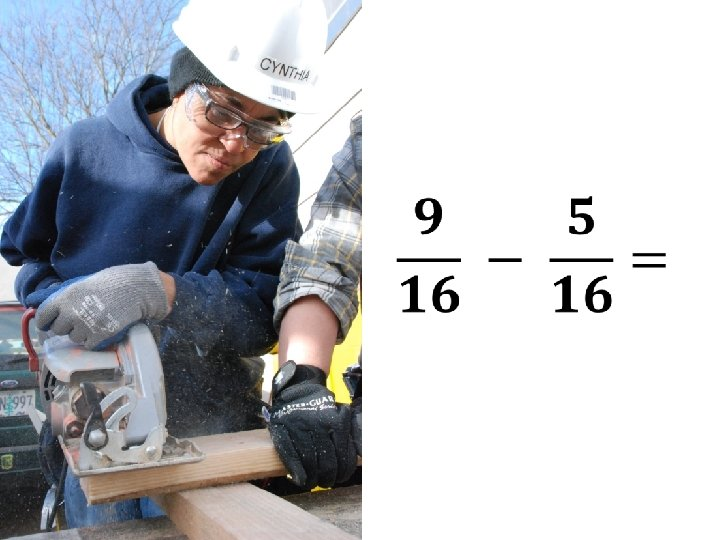When subtracting a fraction from a whole number, borrow a 1 from the whole number, then rename it as a fraction.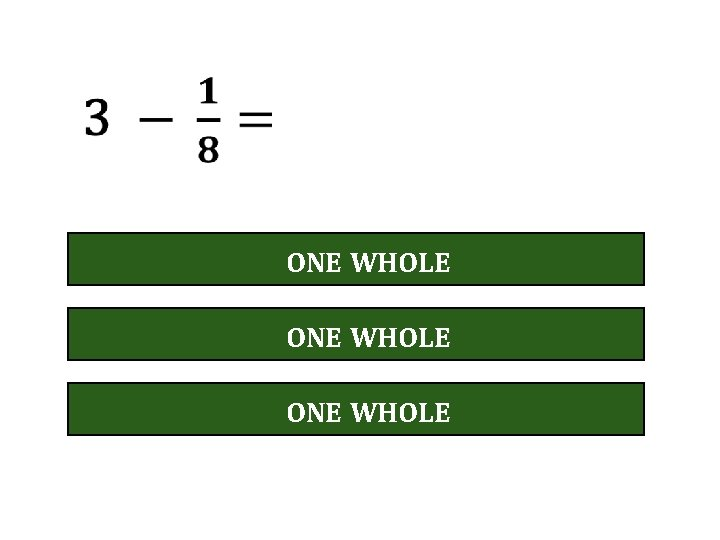ONE WHOLE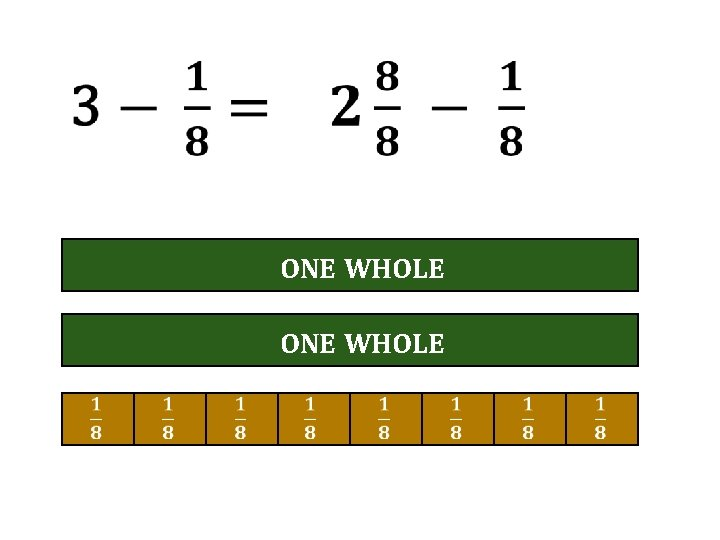ONE WHOLE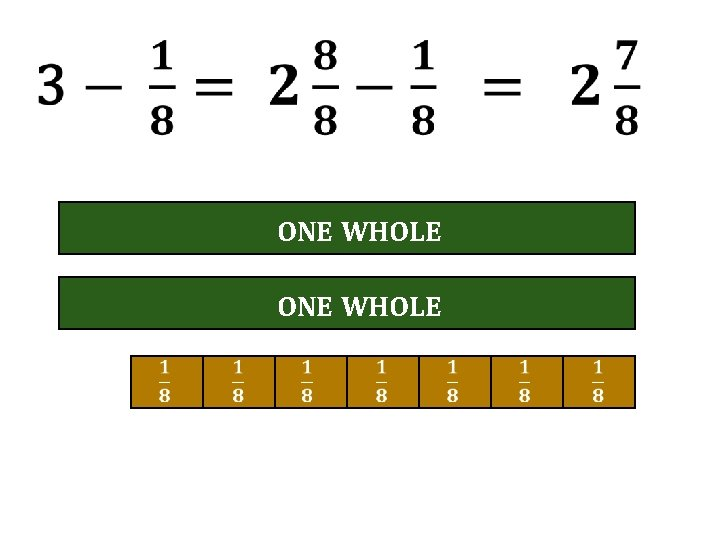ONE WHOLEONE WHOLE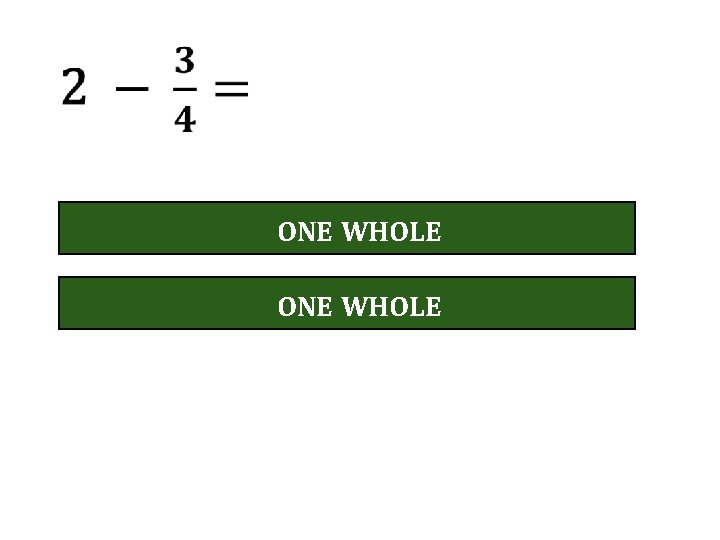ONE WHOLE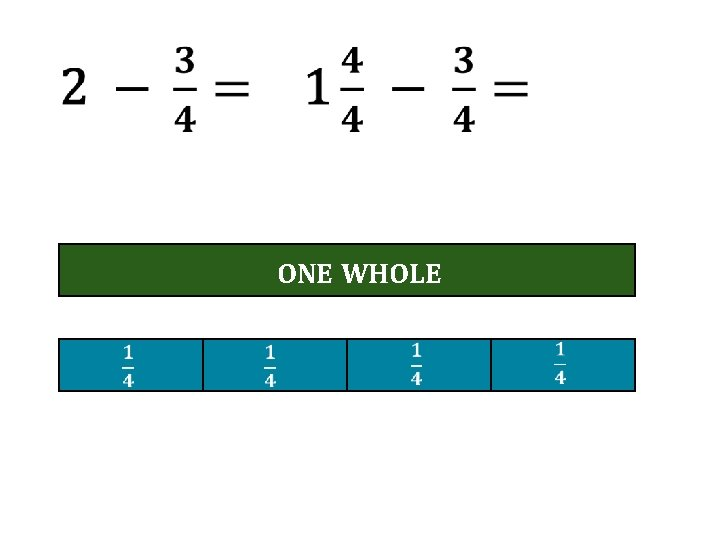ONE WHOLEONE WHOLEWeight in = 45 ¾ tons Weight out = 23 ¼ tonsSubtract whole numbers first, borrow if necessary, subtract the fractions, then combine.Weight in = 45 ¾ tons Weight out = 23 ¼ tons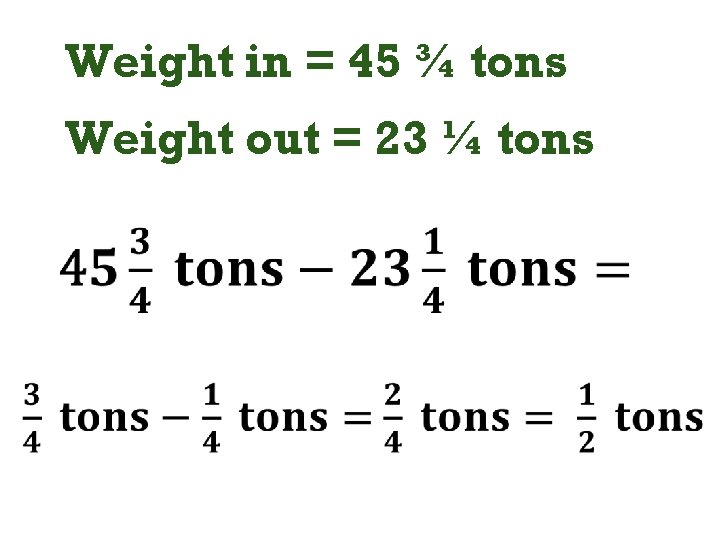Weight in = 45 ¾ tons Weight out = 23 ¼ tonsWeight in = 45 ¾ tons Weight out = 23 ¼ tons Weight of scrap left at the dump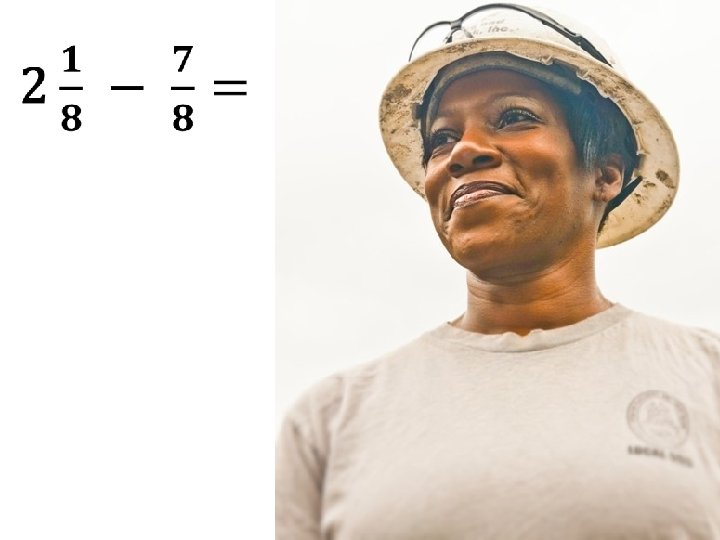ONE WHOLE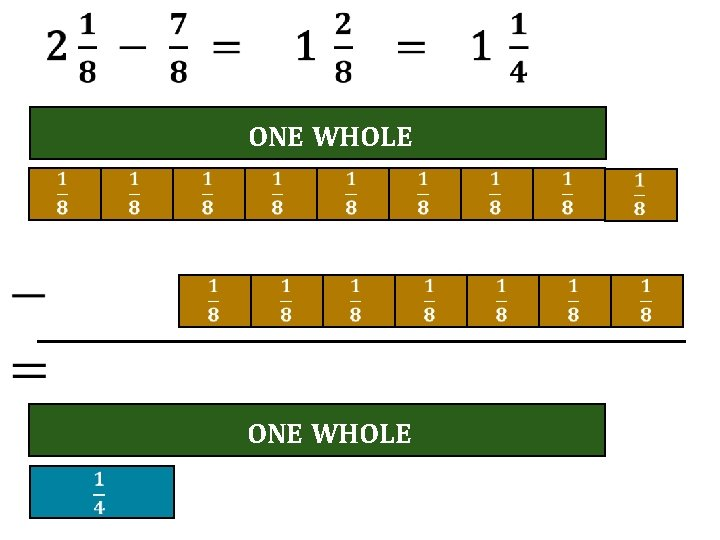ONE WHOLE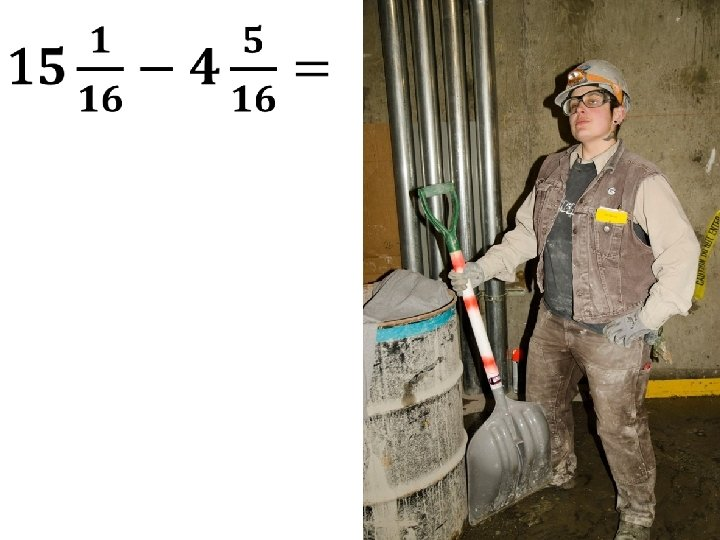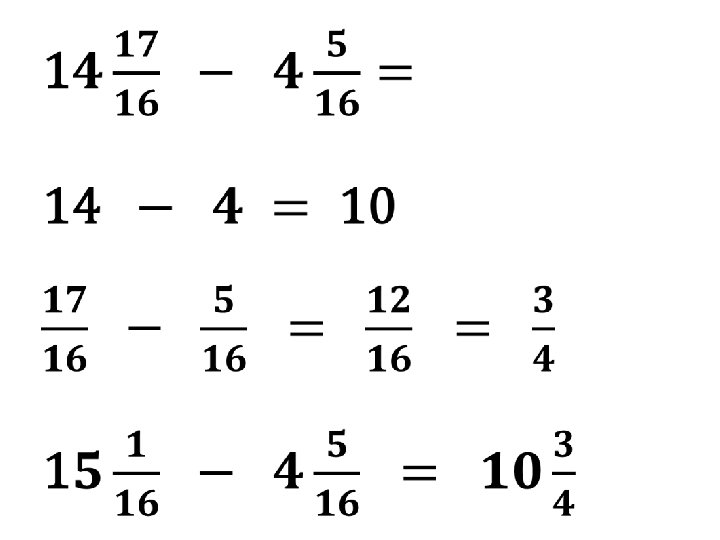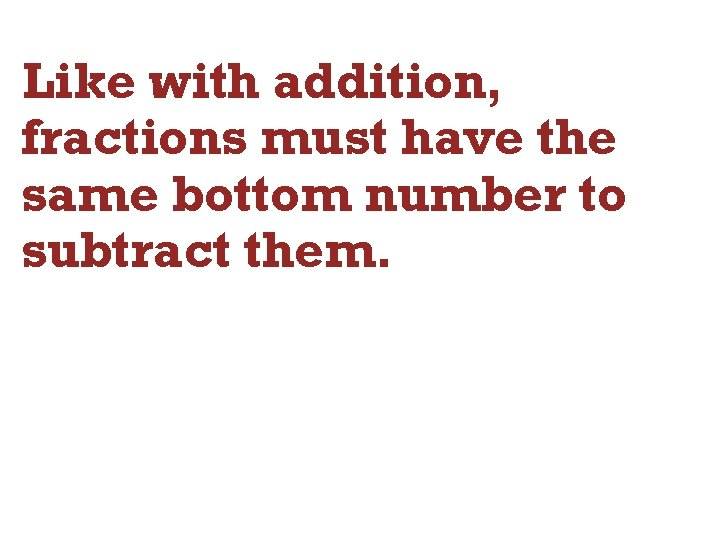Like with addition, fractions must have the same bottom number to subtract them.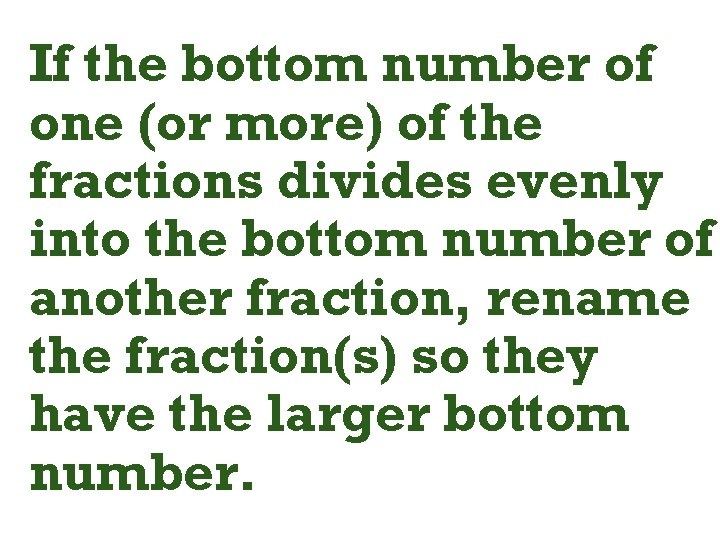If the bottom number of one (or more) of the fractions divides evenly into the bottom number of another fraction, rename the fraction(s) so they have the larger bottom number.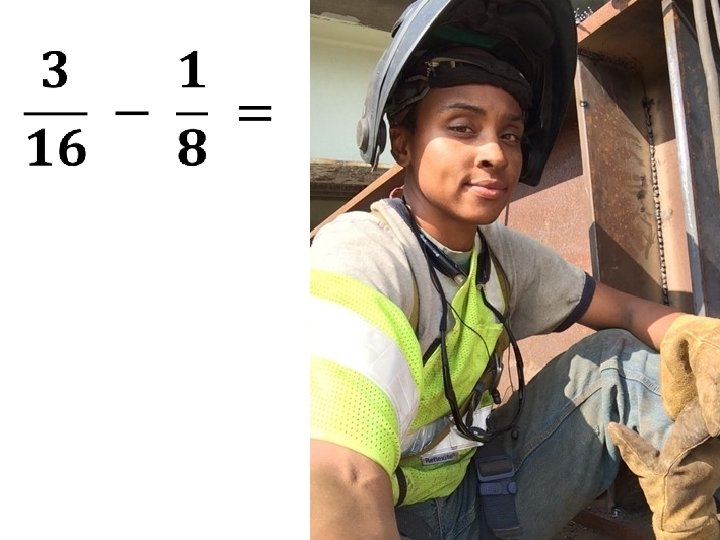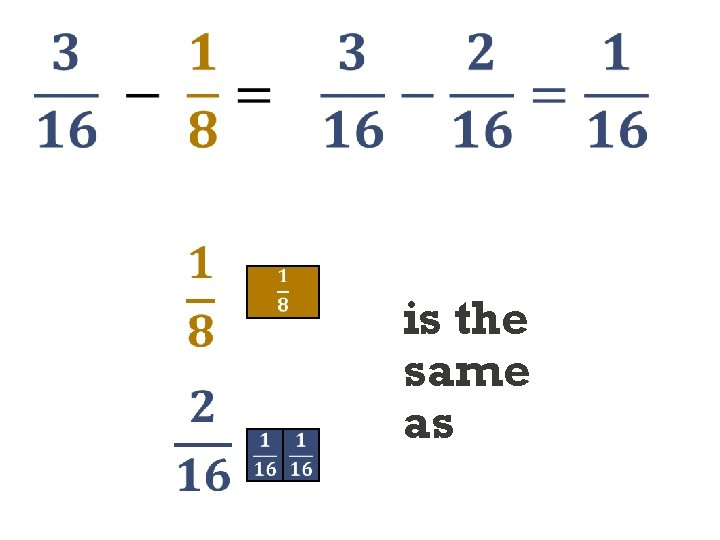is the same as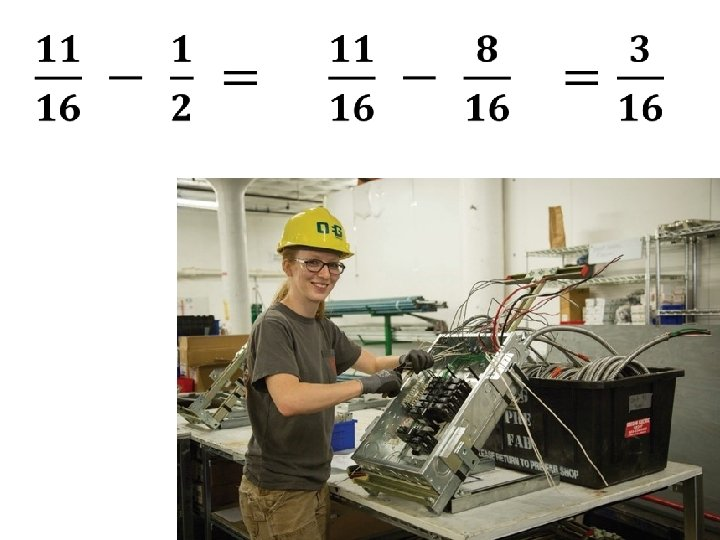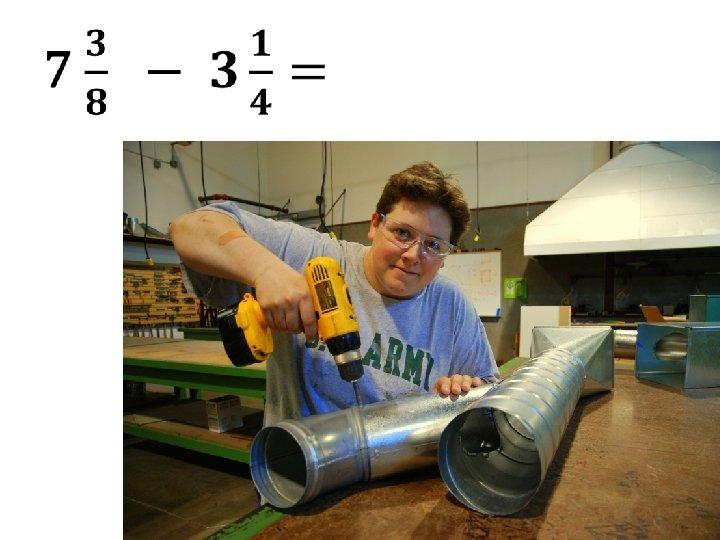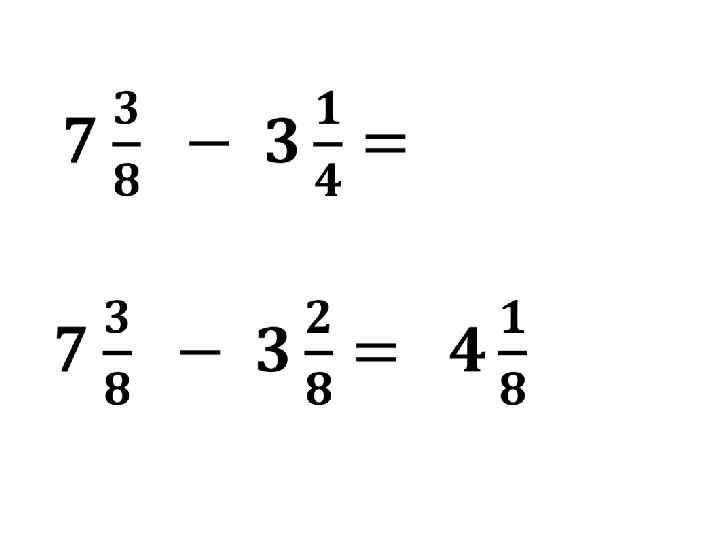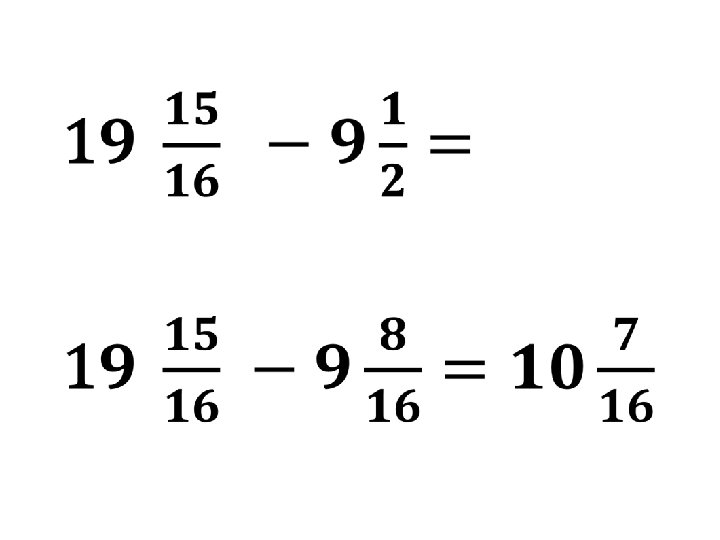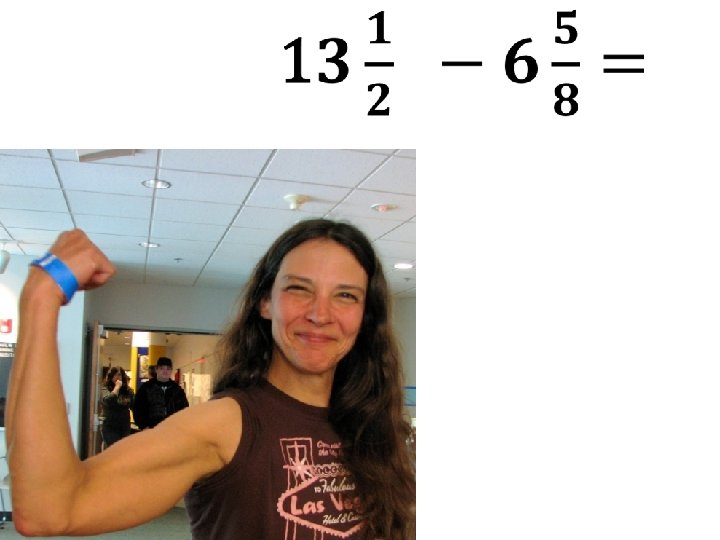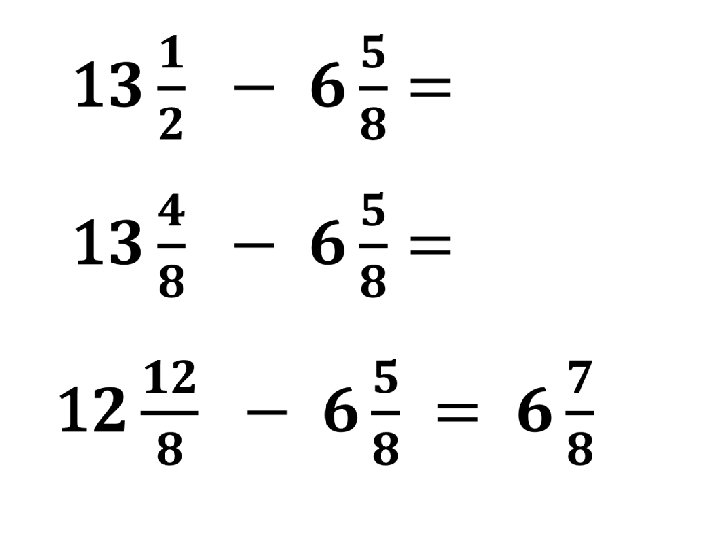You are working on a plumbing job and need a piece of pipe that is. The pipe you have is. How much pipe will be left after you cut the piece you need?Image Credits • Dump Truck 1 b. jpg by John Garrish from Belmont, CA (Dump Truck 1 b) [CC BY-SA 2. 0] via Wikimedia Commons • Lisa. Sawzalls. Portrait 898 A 7268 by Oregon Tradeswomen, Inc. • Tradeswoman by Oregon Tradeswomen, Inc. • Vanessa. Chipping. Wide 898 A 6337 by Oregon Tradeswomen, Inc.